Next: Two-Body Dynamics Up: Planetary Motion Previous: Motion in a Nearly

Exercises

1. Halley's comet has an orbital eccentricity of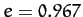and a perihelion distance of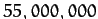miles. Find the orbital period, and the comet's speed at perihelion and aphelion.
2. A comet is first seen at a distance ofastronomical units (1 astronomical unit is the mean Earth-Sun distance) from the Sun, and is traveling with a speed of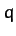times the Earth's mean speed. Show that the orbit of the comet is hyperbolic, parabolic, or elliptical, depending on whether the quantity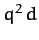is greater than, equal to, or less than 2, respectively.
3. Consider a planet in a Keplerian orbit of major radius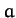and eccentricity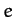about the Sun. Suppose that the eccentricity of the orbit is small (i.e.,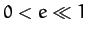), as is indeed the case for all of the planets except Mercury and Pluto. Demonstrate that, to first-order in, the orbit can be approximated as a circle whose center is shifted a distance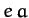from the Sun, and that the planet's angular motion appears uniform when viewed from a point (called the Equant) which is shifted a distance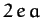from the Sun, in the same direction as the center of the circle. This theorem is the basis of the Ptolomaic model of planetary motion.
4. How long (in days) does it take the Sun-Earth radius vector to rotate through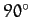, starting at the perihelion point? How long does it take starting at the aphelion point? The period and eccentricity of the Earth's orbit are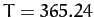days, and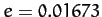, respectively.
5. Solve the Kepler problem for a parabolic orbit to obtain Equations (293)-(295).
6. Solve the Kepler problem for a hyperbolic orbit to obtain Equations (296)-(298).
7. A comet is in a parabolic orbit lying in the plane of the Earth's orbit. Regarding the Earth's orbit as a circle of radius, show that the points where the comet intersects the Earth's orbit are given by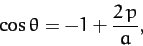where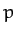is the perihelion distance of the comet, defined at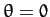. Show that the time interval that the comet remains inside the Earth's orbit is the faction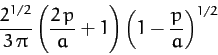of a year, and that the maximum value of this time interval is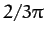year, or about 11 weeks.
8. Prove that in the case of a central force varying inversely as the cube of the distance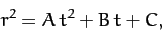where,,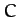are constants.
9. The orbit of a particle moving in a central field is a circle passing through the origin, namely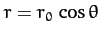. Show that the force law is inverse-fifth power.
10. A particle moving in a central field describes a spiral orbit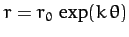. Show that the force law is inverse-cube, and thatvaries logarithmically with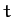. Show that there are two other possible types of orbit in this force-field, and give their equations.
11. A particle moves in a spiral orbit given by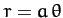. Suppose thatincreases linearly with. Is the force acting on the particle central in nature? If not, determine howwould have to vary within order to make the force central.
12. A particle moves in a circular orbit of radius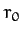in an attractive central force-field of the form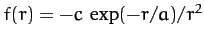, where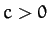and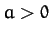. Demonstrate that the orbit is only stable provided that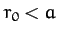.
13. A particle moves in a circular orbit in an attractive central force-field of the form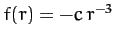, where. Demonstrate that the orbit is unstable to small perturbations.
14. If the Solar System were embedded in a uniform dust cloud, what would the apsidal angle of a planet be for motion in a nearly circular orbit? Express your answer in terms of the ratio of the mass of dust contained in a sphere, centered on the Sun, whose radius is that of the orbit, to the mass of the Sun. This model was once suggested as a possible explanation for the advance of the perihelion of Mercury.

15. The potential energy per unit mass of a particle in the gravitational field of an oblate spheroid, like the Earth, is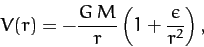where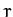refers to distances in the equatorial plane,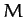is the Earth's mass, and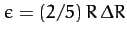. Here,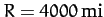is the Earth's equatorial radius, and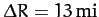the difference between the equatorial and polar radii. Find the apsidal angle for a satellite moving in a nearly circular orbit in the equatorial plane of the Earth.Next: Two-Body Dynamics Up: Planetary Motion Previous: Motion in a Nearly
Richard Fitzpatrick 2011-03-31